Home

# Honest bounds for complexity classes of recursive functions

## Extract

Let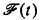be the set of recursive functions computable by machines using at most t(x) computation steps on argument x, for all but finitely many inputs x. We call t a name for the complexity class. Suppose we allow our machines to run longer, say h(x, t(x)) steps on argument x, where h is some fixed recursive function. One might expect that, for large enough h, permitting our machines to run longer by an amount h will always allow us to compute new functions, i.e.,is a proper subset of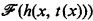. This turns out not to be the case: The “gap theorem” (, ) implies that for every recursive h there exists a recursive t such that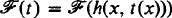. However, if we restrict our attention to names from a certain subclass of the recursive functions, then we can indeed uniformly increase the size of our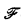-classes. Informally, we call a recursive function t “honest” if some machine computes t(x) in roughly t(x) steps for each argument x. (A precise definition is given in Definition 1 below.) Then according to the “compression theorem” , there exists a single recursive function h such that, for every honest t,is a proper subset of. Thus the phenomenon of the gap theorem is avoided by restricting attention to honest functions. It is a surprising consequence of the “honesty theorem” of McCreight and Meyer (, ) that there is no loss of generality in this restriction. Namely, for any recursive function t there is an honest recursive function t′ such that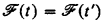.

Hide All

# Honest bounds for complexity classes of recursive functions

## Metrics

### Full text viewsFull text views reflects the number of PDF downloads, PDFs sent to Google Drive, Dropbox and Kindle and HTML full text views.

Total number of HTML views: 0
Total number of PDF views: 0 *Loading metrics...

### Abstract viewsAbstract views reflect the number of visits to the article landing page.

Total abstract views: 0 *Loading metrics...

* Views captured on Cambridge Core between <date>. This data will be updated every 24 hours.

Usage data cannot currently be displayed.